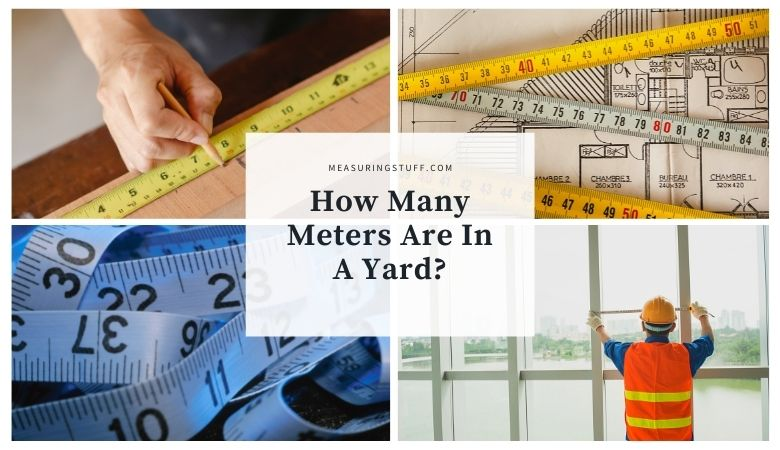# How Many Meters Are In A Yard?

This article will show you how easy it is to convert meters into yards and vice versa.

If you need to know how many meters are in a yard, simply multiply the number of meters by 1.0936 yards to get the answer.

For example, 1 meter x 1.0936 = 1.0936 yards and 5 meters x 1.0936 = 5.468 yards.

If you want to figure out how many yards are in a meter, multiply the number of yards by 0.9144.

For example, 1 yard x 0.9144 = 0.9144 meters and 5 yards x 0.9144 = 4.572 meters.

Of course, this is great if you have a calculator handy or can do the math in your head.

If you prefer, you can convert between meters and yards by using a conversion table or conversion calculator.

Check out the following tables and calculator.

## Meters And Yards Measurements

A Meter is part of the metric system of measurement which was created in France to have a way to measure length, mass, and volume. 1 meter is equal to 3 feet 3 inches in length.

A yard is also a length unit of measurement used by both the British imperial and US customary systems of measurement.

1 yard is equal to 3 feet or 36 inches. An international yard is equal to 0.9144 inches.

The term yard can be abbreviated with the letters yd, for example 1 yard can be written as 1 yd.

## Meters To Yards Conversion Calculator

Use the following calculator to easily convert meters into yards.

For our complete section of unit conversions including tables and calculators click the link here.